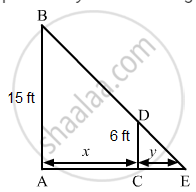PUC Karnataka Science Class 12Department of Pre-University Education, Karnataka
Share

A Man of Height 6 Ft Walks at a Uniform Speed of 9 Ft/Sec from a Lamp Fixed at 15 Ft Height. the Length of His Shadow is Increasing at the Rate of (A) 15 Ft/Sec (B) 9 Ft/Sec - PUC Karnataka Science Class 12 - Mathematics

Question

A man of height 6 ft walks at a uniform speed of 9 ft/sec from a lamp fixed at 15 ft height. The length of his shadow is increasing at the rate of

• 15 ft/sec

• 9 ft/sec

•  6 ft/sec

• none of these

Solution

6 ft/sec
Let AB be the lamp post. Suppose at any time t, the man CD be at a distance of x km from the lamp post and y ft be the length of his shadow CE.$\text { Since the triangles ABE and CDE are similar },$

$\frac{AB}{CD} = \frac{AE}{CE}$

$\Rightarrow \frac{15}{6} = \frac{x + y}{y}$

$\Rightarrow \frac{x}{y} = \frac{15}{6} - 1$

$\Rightarrow \frac{x}{y} = \frac{3}{2}$

$\Rightarrow y = \frac{2}{3}x$

$\Rightarrow \frac{dy}{dt} = \frac{2}{3}\left( \frac{dx}{dt} \right)$

$\Rightarrow \frac{dy}{dt} = \frac{2}{3} \times 9$

$\Rightarrow \frac{dy}{dt} = 6 \text { ft }/\sec$

Is there an error in this question or solution?

Video TutorialsVIEW ALL 

Solution A Man of Height 6 Ft Walks at a Uniform Speed of 9 Ft/Sec from a Lamp Fixed at 15 Ft Height. the Length of His Shadow is Increasing at the Rate of (A) 15 Ft/Sec (B) 9 Ft/Sec Concept: Rate of Change of Bodies Or Quantities.
S